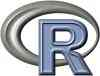## Statistical Programming with R, the Analytics way

• 20 September 2014 to 21 September 2014

This is a 2 full days event. Limited 30 seats only. Seats would be confirmed on FIRST-CUM-FIRST serve basis, and  payment is received.

In this course you will learn how to program in R and how to use R for effective data analysis. You will learn how to install and configure R software for a statistical programming environment and describe generic programming language concepts as they are implemented in a high-level statistical language. The course covers practical issues in statistical computing which includes programming in R, reading data into R, accessing R packages, writing R functions, debugging, profiling R code, and organizing and commenting R code. Topics in statistical data analysis will provide working examples.

` Program Objectives: • Master the use of R Console • Learn R flow control and data structures • Use vectorized calculations • Write R functions • Understand basic R graphics • Familiar with advanced graphics • Discover how to install R packages • Explore the R documentation • Learn to avoid R pitfalls • Use R for descriptive statistics • Use R for inferential statistics • Write R statistical models`